• 整型数字转字符(包括负数)  */ #include #include /* 判断这个数字是几位数  */ int num_length(int num) { int length=0; if(num { num*=-1; while(num) { num=num/10; length++; } return length+1; }
/*
整型数字转字符(包括负数)
*/
#include<stdio.h>
#include<stdlib.h>
/*
判断这个数字是几位数
*/
int num_length(int num)
{
int length=0;
if(num<0)
{
num*=-1;
while(num)
{
num=num/10;
length++;
}
return length+1;

}
else
{
while(num)
{
num=num/10;
length++;
}

return length;
}

}
/*
把数字进行拆分存入一个字符数组中
*/
char *itoa(int num)
{
int i,pos=0;
int length;
char *str = (char *)malloc(num_length(num)*sizeof(char)+1);
if(str==NULL)
{
printf("内存申请失败！");
}
length = num_length(num);   //返回整型数字的位数
/* 1.0  这里遇到了一个问题，就是局部变量 str[length+1] (数组)
在函数结束时即使返回了数组的首地址，即使首地址有效，
但是里面的已经没有意义了，所以在主函数调用时，
输出的内容是乱码，定义成全局变量后解决了这个问题
statci 无法给长度会变化的数组变量进行修饰

2.0 经过测试使用malloc来进行内存的申请一段连续的内存，
然后将转换后的字符存入这个连续的内存中
*/
//算法：从高位到低位取数  除10取模从高地址到低地址存存到malloc开辟的内存中

pos=length;
*(str+pos)='\0';    //地址最高赋值\0,字符串的最后要加上\0
pos--;
if(num<0)          //如果是负数
{
num*=-1;      //*-1转换成正整数方便拆分
while(num)
{
*(str+pos)=(num%10)+'0';  //数字转成字符+'0'
num=num/10;
pos--;

}

*str='-';        //最低位，也就是起始地址赋值'负号 '

}
else
{
while(num)
{
*(str+pos)=(num%10)+'0';  //数字转成字符+'0'
num=num/10;
pos--;

}
}

return str;

}
int main(void)
{
int number=0;

char *p;

scanf("%d",&number);
printf("%s",itoa(number));
=pitoa(number);
free(p);//释放申请的内存空间
p=NULL;             //指针赋值为NULL防止野指针的出现

return 0;
}
展开全文• #include #include void to_str(n) int n; { char s; int i = 0; if(n) { putchar('-'); n = -n; } do { s[i++] = n%10 +'0'; n/=10; } while(n>0); while(i--)putchar(s[i]);...c
• #include void itoa(int ,char *);.../*itoa 函数： 将数字转换成字符串并保存到S中*/ void itoa(int n, char s[]) { int i,sign; if((sign = n) ) n = -n; i = 0; do { s[i++] = n % 10
#include <stdio.h>

void itoa(int ,char *);
void reverse(char *);

/*itoa 函数： 将数字转换成字符串并保存到S中*/
void itoa(int n, char s[])
{
int i,sign;

if((sign = n) < 0)
n = -n;
i = 0;
do
{
s[i++] = n % 10 + '0';
}while((n /= 10) > 0);

if(sign < 0)
s[i++] = '-';
s[i] = '\0';

reverse(s);

}

/*reverse 函数：字符串反转（指针方法不安全）*/
void reverse(char *s)
{
int len = strlen(s);

char *ptr1,*ptr2;

ptr1 = s;
ptr2 = s + len - 1;

while(ptr1 != ptr2)
{
char temp;
temp = *ptr1;
*ptr1 = *ptr2;
*ptr2 = temp;
ptr1++;
if(ptr1 == ptr2)
break;
else
ptr2--;
}

}

/*《The C programming language(second edition)》中实现int型转字符串练习*/
int main()
{ int a;
char s;
scanf("%d",&a);
itoa(a,s);
printf("The int is %s\n",s);

return 0;
}


展开全文itoa
• ## C语言整数与字符串的相互转换

万次阅读 多人点赞 2018-06-26 23:22:57
C语言 整数字符串的相互转换 ...二、整数转字符串  1、拓展函数itoa  itoa (表示 integer to alphanumeric)是把整型数转换成字符串的一个函数。  windows环境下，在&lt;stdlib.h&gt;头文件中有 c...
C语言 整数与字符串的相互转换

一、简述

C语言中整数与字符串的相互转换，有广泛应用的拓展函数(非标准库)，也可以自己尝试简单的实现。

二、整数转字符串

1、拓展函数itoa

itoa (表示 integer to alphanumeric)是把整型数转换成字符串的一个函数。

windows环境下，在<stdlib.h>头文件中有

char* itoa(int value,char*string,int radix);//value: 要转换的整数，string: 转换后的字符串,radix: 转换进制数，如2,8,10,16 进制等。

函数源码：

char* itoa(int num,char* str,int radix)
{
char index[]="0123456789ABCDEFGHIJKLMNOPQRSTUVWXYZ";//索引表
unsigned unum;//存放要转换的整数的绝对值,转换的整数可能是负数
int i=0,j,k;//i用来指示设置字符串相应位，转换之后i其实就是字符串的长度；转换后顺序是逆序的，有正负的情况，k用来指示调整顺序的开始位置;j用来指示调整顺序时的交换。

//获取要转换的整数的绝对值
{
unum=(unsigned)-num;//将num的绝对值赋给unum
str[i++]='-';//在字符串最前面设置为'-'号，并且索引加1
}
else unum=(unsigned)num;//若是num为正，直接赋值给unum

//转换部分，注意转换后是逆序的
do
{

}while(unum);//直至unum为0退出循环

str[i]='\0';//在字符串最后添加'\0'字符，c语言字符串以'\0'结束。

//将顺序调整过来
if(str=='-') k=1;//如果是负数，符号不用调整，从符号后面开始调整
else k=0;//不是负数，全部都要调整

char temp;//临时变量，交换两个值时用到
for(j=k;j<=(i-1)/2;j++)//头尾一一对称交换，i其实就是字符串的长度，索引最大值比长度少1
{
temp=str[j];//头部赋值给临时变量
str[j]=str[i-1+k-j];//尾部赋值给头部
str[i-1+k-j]=temp;//将临时变量的值(其实就是之前的头部值)赋给尾部
}

return str;//返回转换后的字符串
}

示例程序：

#include <stdlib.h>
#include <stdio.h>
int main()
{
int number1 = 123456;
int number2 = -123456;
char string = {0};
itoa(number1,string,10);
printf("数字：%d 转换后的字符串为：%s\n",number1,string);
itoa(number2,string,10);
printf("数字：%d 转换后的字符串为：%s\n",number2,string);
return 0;
}

效果截图：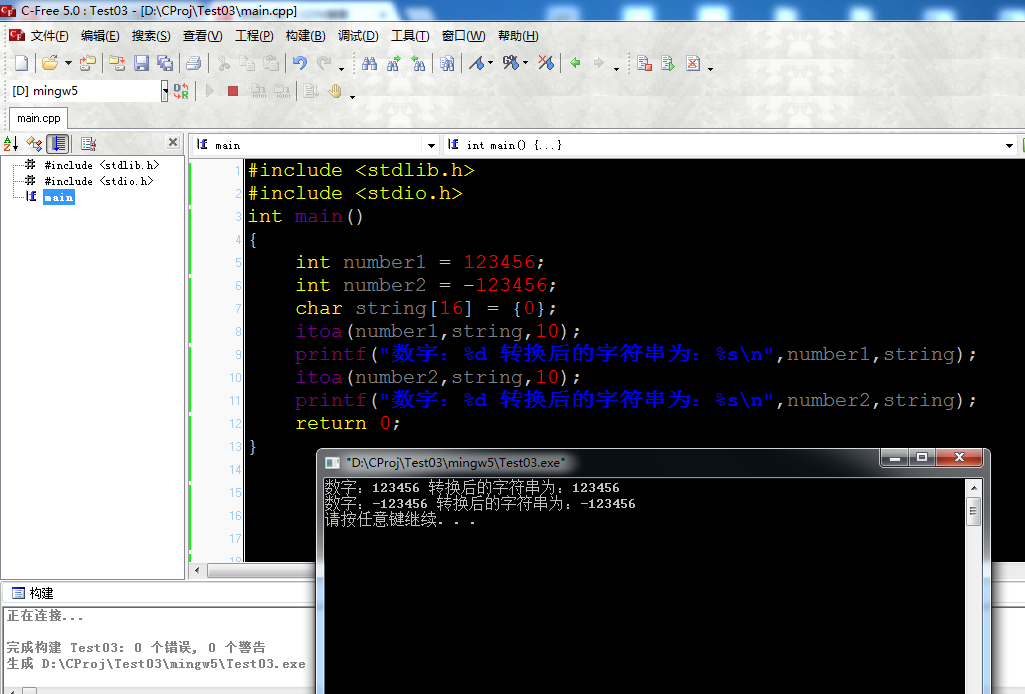2、自己简单实现

实现代码


#include <stdio.h>

char* Int2String(int num,char *str);//函数声明
int main()
{
int number1 = 123456;
int number2 = -123456;
char string = {0};
Int2String(number1,string);
printf("数字：%d 转换后的字符串为：%s\n",number1,string);
Int2String(number2,string);
printf("数字：%d 转换后的字符串为：%s\n",number2,string);
return 0;
}

char* Int2String(int num,char *str)//10进制
{
int i = 0;//指示填充str
if(num<0)//如果num为负数，将num变正
{
num = -num;
str[i++] = '-';
}
//转换
do
{
str[i++] = num%10+48;//取num最低位 字符0~9的ASCII码是48~57；简单来说数字0+48=48，ASCII码对应字符'0'
num /= 10;//去掉最低位
}while(num);//num不为0继续循环

str[i] = '\0';

//确定开始调整的位置
int j = 0;
if(str=='-')//如果有负号，负号不用调整
{
j = 1;//从第二位开始调整
++i;//由于有负号，所以交换的对称轴也要后移1位
}
//对称交换
for(;j<i/2;j++)
{
//对称交换两端的值 其实就是省下中间变量交换a+b的值：a=a+b;b=a-b;a=a-b;
str[j] = str[j] + str[i-1-j];
str[i-1-j] = str[j] - str[i-1-j];
str[j] = str[j] - str[i-1-j];
}

return str;//返回转换后的值
}

效果截图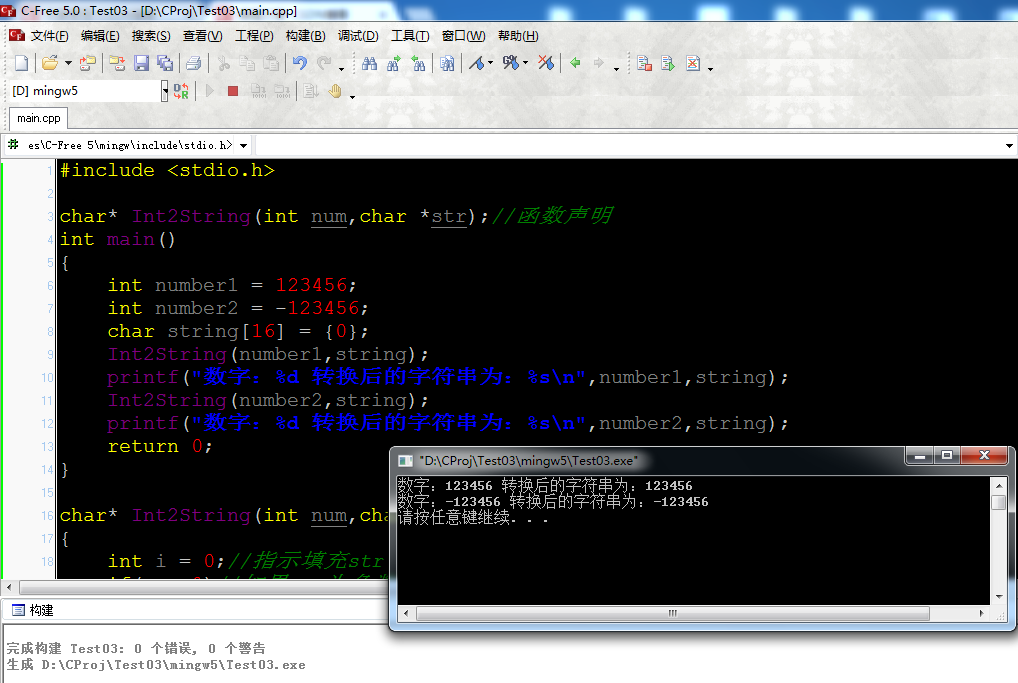三、字符串转整数

1、拓展函数
atoi (表示 alphanumeric to integer)是把字符串转换成整型数的一个函数。
windows环境下，在<stdlib.h>头文件中

int  atoi(const char *nptr);//字符串转整数函数，nptr: 要转换的字符串

源码：

int  atoi(const char *nptr)
{
return (int)atol(nptr);
}

long  atol(const char *nptr)
{
int c;              /* 当前要转换的字符(一个一个字符转换成数字) */
long total;         /* 当前转换结果 */
int sign;           /* 标志转换结果是否带负号*/

/*跳过空格，空格不进行转换*/
while ( isspace((int)(unsigned char)*nptr) )
++nptr;

c = (int)(unsigned char)*nptr++;//获取一个字符准备转换
sign = c;           /*保存符号标示*/
if (c == '-' || c == '+')
c = (int)(unsigned char)*nptr++;    /*跳过'+'、'-'号，不进行转换*/

total = 0;//设置转换结果为0

while (isdigit(c)) {//如果字符是数字
total = 10 * total + (c - '0');     /* 根据ASCII码将字符转换为对应的数字，并且乘10累积到结果 */
c = (int)(unsigned char)*nptr++;    /* 取下一个字符 */
}

//根据符号指示返回是否带负号的结果
if (sign == '-')
return -total;
else
}

示例程序：

#include<stdio.h>
#include<stdlib.h>

int main()
{
printf("字符串\"123456\"转换为数字：%d\n",atoi("123456"));
printf("字符串\"-123456\"转换为数字：%d\n",atoi("-123456"));
return 0;
}

效果截图：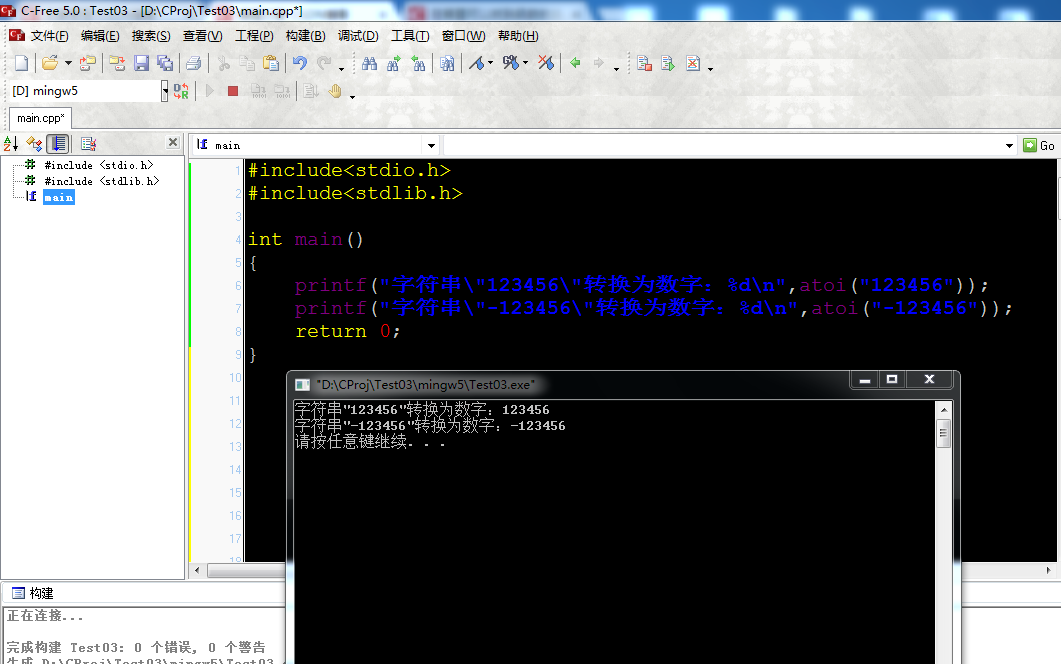2、自己简单实现

实现源码

#include<stdio.h>

int String2Int(char *str);//函数声明
int main()
{
printf("字符串\"123456\"转换为数字：%d\n",String2Int("123456"));
printf("字符串\"-123456\"转换为数字：%d\n",String2Int("-123456"));
return 0;
}

int String2Int(char *str)//字符串转数字
{
char flag = '+';//指示结果是否带符号
long res = 0;

if(*str=='-')//字符串带负号
{
++str;//指向下一个字符
flag = '-';//将标志设为负号
}
//逐个字符转换，并累加到结果res
while(*str>=48 && *str<57)//如果是数字才进行转换，数字0~9的ASCII码：48~57
{
res = 10*res+  *str++-48;//字符'0'的ASCII码为48,48-48=0刚好转化为数字0
}

if(flag == '-')//处理是负数的情况
{
res = -res;
}

return (int)res;
}


截图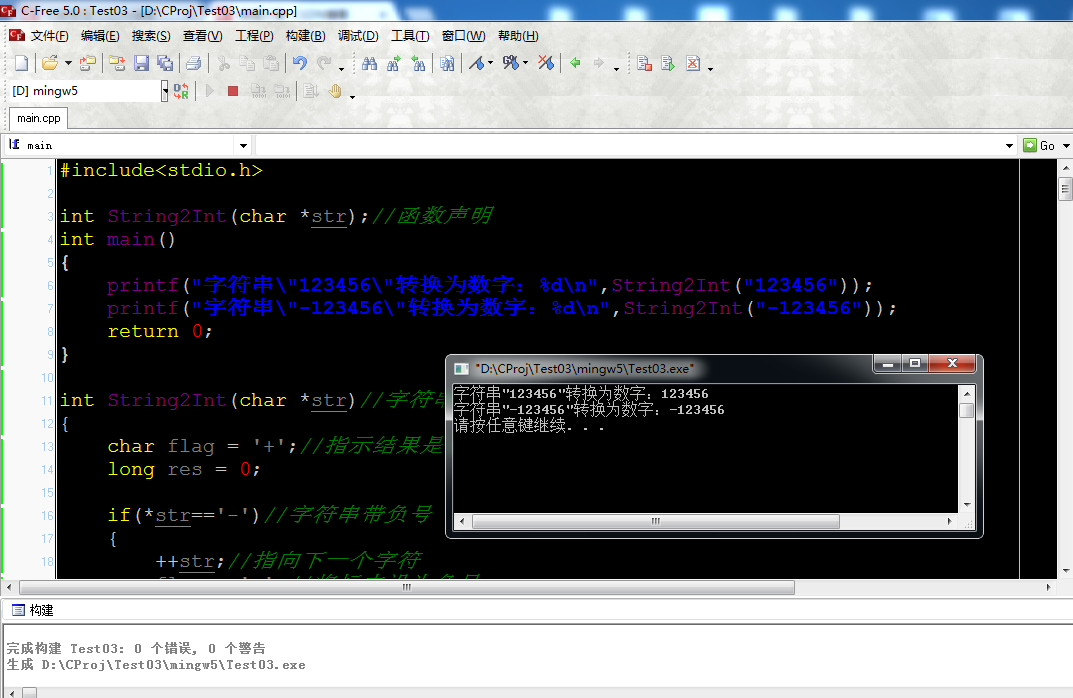四、利用sprintf()函数和sscanf()函数

整数转字符串

测试代码：

#include <stdio.h>

char* Int2String(int num,char *str);//函数声明
int main()
{
int number1 = 123456;
int number2 = -123456;
char string = {0};
Int2String(number1,string);
printf("数字：%d 转换后的字符串为：%s\n",number1,string);
Int2String(number2,string);
printf("数字：%d 转换后的字符串为：%s\n",number2,string);
return 0;
}

char* Int2String(int num,char *str)
{
sprintf(str, "%d", num);

return str;

}


运行结果：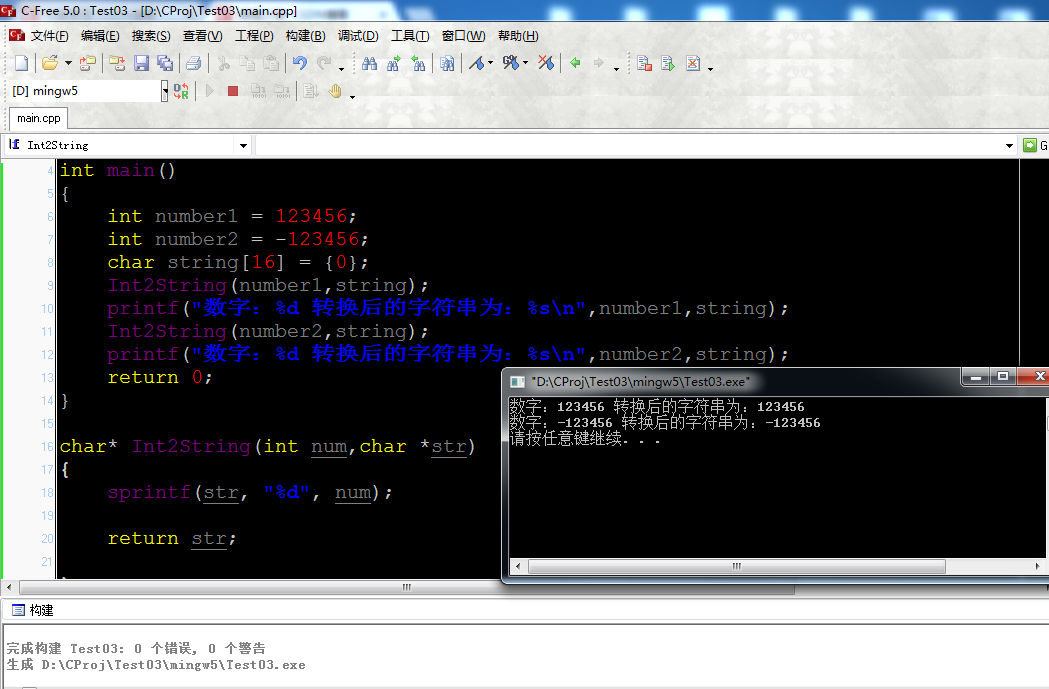字符串转整数

测试代码：

#include<stdio.h>

int String2Int(char *str);//函数声明
int main()
{
printf("字符串\"123456\"转换为数字：%d\n",String2Int("123456"));
printf("字符串\"-123456\"转换为数字：%d\n",String2Int("-123456"));
return 0;
}

int String2Int(char *str)//字符串转数字
{
char flag = '+';//指示结果是否带符号
long res = 0;

if(*str=='-')//字符串带负号
{
++str;//指向下一个字符
flag = '-';//将标志设为负号
}

sscanf(str, "%ld", &res);
if(flag == '-')
{
res = -res;
}
return (int)res;
}

运行结果：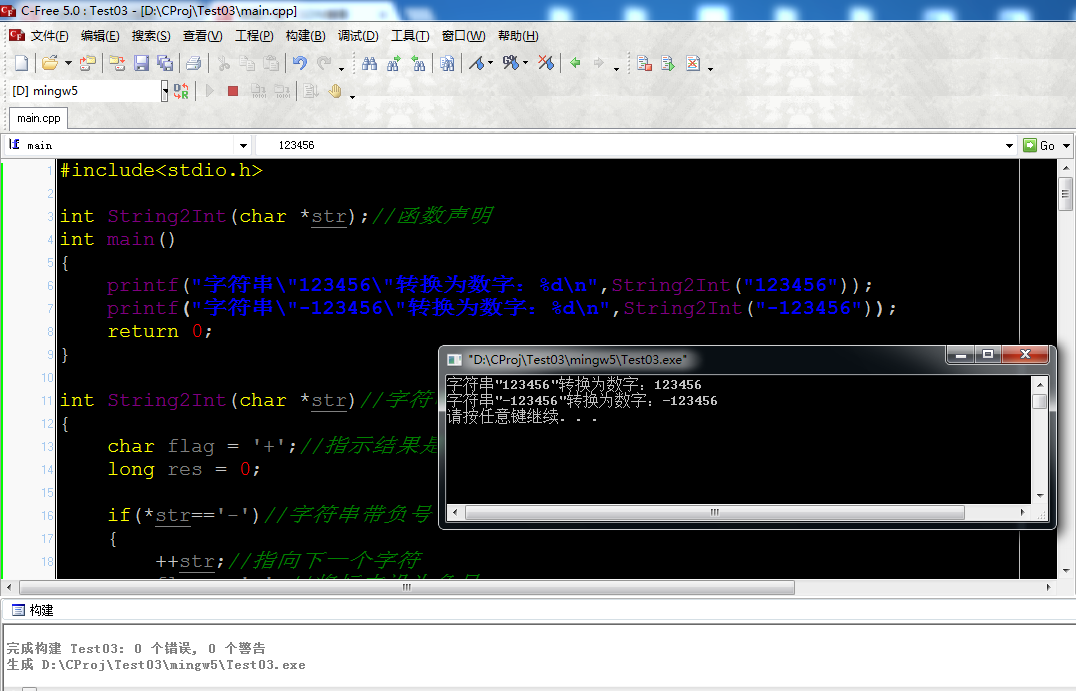五、附ASCII码表(部分)

ASCII值
控制字符
ASCII值
控制字符
ASCII值
控制字符
ASCII值
控制字符
0
NUT
32
(space)
64
@
96
、
1
SOH
33
!
65
A
97
a
2
STX
34
"
66
B
98
b
3
ETX
35
#
67
C
99
c
4
EOT
36
\$
68
D
100
d
5
ENQ
37
%
69
E
101
e
6
ACK
38
&
70
F
102
f
7
BEL
39
,
71
G
103
g
8
BS
40
(
72
H
104
h
9
HT
41
)
73
I
105
i
10
LF
42
*
74
J
106
j
11
VT
43
+
75
K
107
k
12
FF
44
,
76
L
108
l
13
CR
45
-
77
M
109
m
14
SO
46
.
78
N
110
n
15
SI
47
/
79
O
111
o
16
DLE
48
0
80
P
112
p
17
DCI
49
1
81
Q
113
q
18
DC2
50
2
82
R
114
r
19
DC3
51
3
83
S
115
s
20
DC4
52
4
84
T
116
t
21
NAK
53
5
85
U
117
u
22
SYN
54
6
86
V
118
v
23
TB
55
7
87
W
119
w
24
CAN
56
8
88
X
120
x
25
EM
57
9
89
Y
121
y
26
SUB
58
:
90
Z
122
z
27
ESC
59
;
91
[
123
{
28
FS
60
<
92
/
124
|
29
GS
61
=
93
]
125
}
30
RS
62
>
94
^
126

31
US
63
?
95
_
127
DEL

展开全文• C语言整数转换字符的方法 char* int_to_change(int i) { int j,buf,a; char* buffer; while(1) { j = i%10; j += 48; buffer[a] = j; i = i/10; a++; if(j == 0) break; } return buffer; }
C语言整数转换字符的方法
char* int_to_change(int i)
{
int j,buf,a;
char* buffer;
while(1)
{
j =  i%10;
j += 48;
buffer[a] = j;
i = i/10;
a++;
if(j == 0)
break;
}
return buffer;
}

`
展开全文• C语言提供了几个标准库函数，可以将任意类型(整型、长整型、浮点型等)的数字转换为字符串。 1.int/float to string/array: C语言提供了几个标准库函数，可以将任意类型(整型、长整型、浮点型等)的数字转换为...
• //最后还得把前面的字符串的i位清零  return ;  }  char c = (n % 10) + '0';  int i = strlen(str);  str[i] = c;  i++;  str[i] = 0;   } int convert2(char str[]) {  if('-' = str) ...
• C语言itoa()函数和atoi()函数详解(整数转字符C实现) C语言提供了几个标准库函数，可以将任意类型(整型、长整型、浮点型等)的数字转换为字符串。 1.int/float to string/array: C语言提供了几个标准库函数，可以将...
• 整数字符串变数字 */ #include #include #include int strToNumber(); int powTen(int n);//返回10的n次方 void main() { printf("你输入的整数位%d\n",strToNumber());//读入整数字符串，返回int整数 } int powTen...
• 1（字符1） 函数返回后，12 123 1234 又依次出栈 回答 首先，1没有入栈 我们把convert()函数当作一个运算来描述（实际上它就是一种计算机运算） 运算convert(1234)时，程序运行到if语句时，会再次转入convert()...递归
• 逆序将整数单个位数转换成字符赋值进入数组，此时得到一个逆序的字符串数组，最后要加’\0’结束符； 输出时逆序输出，或者逆置字符串数组再输出也可以得到结果； #include<stdio.h> #include<string.h>...
• c语言中将整数转换成字符串Given an ASCII string (char[]) and we have to convert it into Hexadecimal string (char[]) in C. 给定一个ASCII字符串(char [])，我们必须在C中将其转换为十六进制字符串(char [])。...python java 算法
• C语言将正整数转换为字符C语言将正整数转换为字符串完整源码 C语言将正整数转换为字符串完整源码 #include <assert.h> #include <inttypes.h> #include <stdio.h> #include <stdlib.h> #...
• ## C语言整数

千次阅读 2019-08-03 20:10:15
整数是我们生活中常用的数据类型，也是编程中常用的一种数据，C语言使用int关键字来定义整数变量（int 是 integer 的简写）。 在定义变量的时候，可以加signed、unsigned、short和long四种修饰符。 s...C++语言 int integer
• c语言中将整数转换成字符串Given an ASCII string (char[]) and we have to convert it into octal string (char[]) in C. 给定一个ASCII字符串(char [])，我们必须在C中将其转换为八进制字符串(char [])。 Logic:...
• #include&amp;amp;amp;amp;lt;stdio.h&amp;amp;amp;amp;gt; void main() { int num=12345; int i=0,j=0; char temp,str; printf(&amp;... //输出原整数 while(num) { te
• C语言itoa()函数和atoi()函数详解(整数转字符C实现) 社区：博客园 作者：petercao 博文：C语言itoa()函数和atoi()函数详解(整数转字符C实现) 网址：http://www.cnblogs.com/bluestorm/p/3168719.html C语言...
• C语言提供了几个标准库函数，可以将任意类型(整型、长整型、浮点型等)的数字转换为字符串。 1.int/float to string/array: C语言提供了几个标准库函数，可以将任意类型(整型、长整型、浮点型等)的数字转换为字符串...
• atof（将字符串转换成浮点型数） 表头文件 #include <stdlib.h> 定义函数 double atof(const char nptr); 函数说明 atof()会扫描参数nptr字符串，跳过前面的空格字符，直到遇上数字或正负符号才开始做转换，而...
• 一，atoi()函数——将字符串str转换成一个整数 1、atoi函数把字符串转换成整型数。其含义是ASCII to integer 的缩写。 2、函数说明 1）简介编辑C语言库函数名 atoi 原型： int atoi(const char *nptr); ...
• 文章目录1、把整数/长整数格式化输出到字符串2、注意事项3、版权声明 各位可能在网上看到用以下函数可以将整数转换为字符串： itoa(); //将整型值转换为字符串 ultoa(); // 将无符号长整型值转换为字符串 请注意...
• C语言实现整数转化为字符串 转化函数 整数转化为字符串 char *int_to_str(int x, char *str) { char ch, *p; int sign = 0, r; p = str; if (x < 0) { //处理负数 sign = 1; x = -x; } while (x > ...
• c语言scanf输入字符We have to read tree values: integer, float and then character using only one scanf() function and then print all values in separate lines. 我们只需要使用一个scanf()函数读取树值：...python java 算法 linux...

# c语言整数转字符c语言 订阅